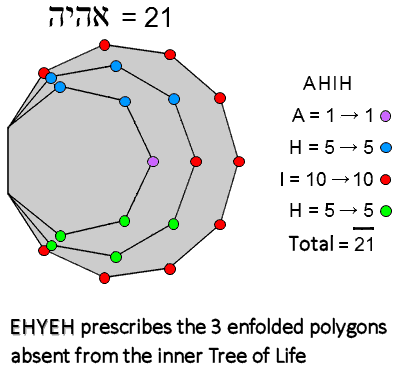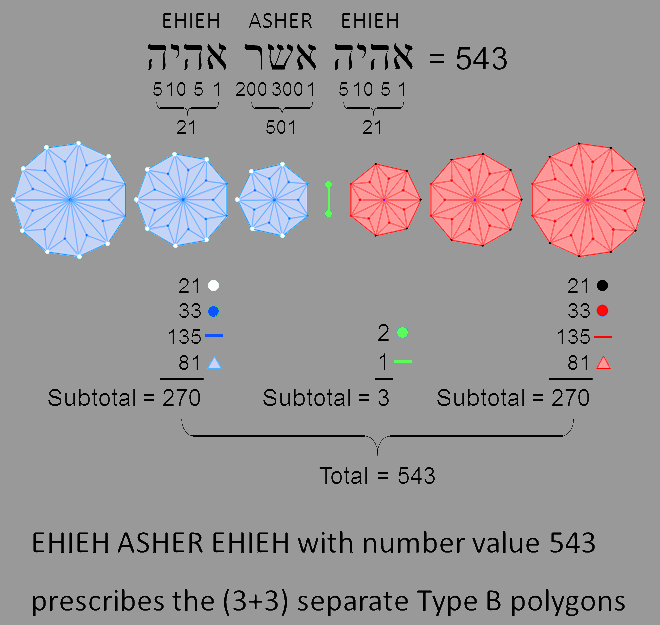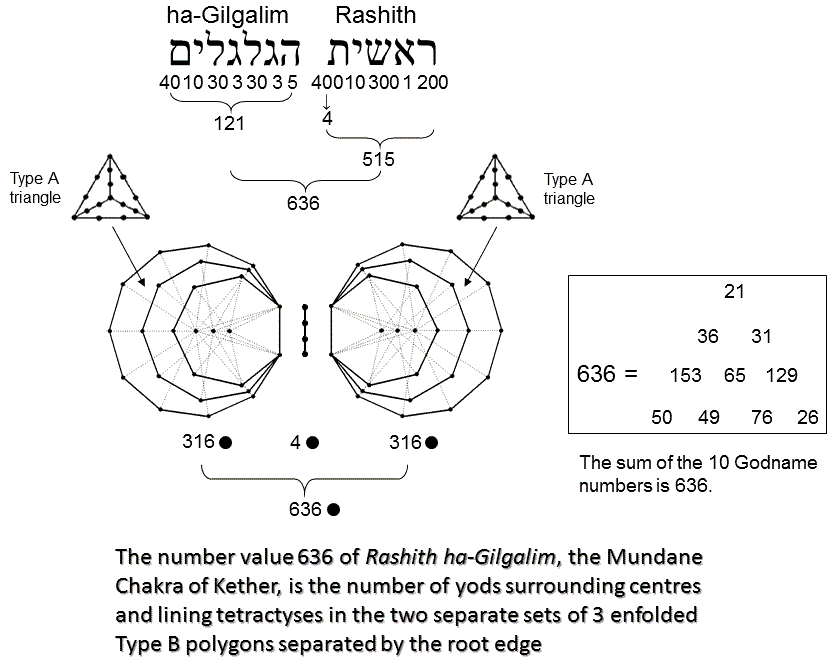<< Previous    1  2    4  5  ...17    Next >>

KetherThe 3 enfolded Type A polygons have 21 corners outside their root edge that surround their centres. This is the number value of EHIEH ("I am"), the Godname of Kether. Its letter values are the number of sets of yods symmetrically arranged about the horizontal axis. These 21 corners create the shapes of the 3 enfolded polygons as they grow out of the root edge. They amount to the minimal amount of information needed to create the boundary, or skeletal form, of the polygons before their division into simple, triangular sectors (1st-order polygons, or Type A) and higher-order triangles. This minimal character is consistent with Kether (the first Sephirah) as the seed source of the Tree of Life. Notice that the number 21 appears in some of the formulae shown above for the various geometrical and yod populations of the 3 enfolded, nth-order polygons.According to Table 2, the number of geometrical elements in the 3 separate Type B polygons = G2 = 273. Hence, 270 geometrical elements surround their centres. They comprise: 21 polygonal corners outside the sides that become the root edge when they are enfolded; 33 corners that are either endpoints of the sides that become the root edge or internal corners of triangles; (5×27=135) sides; (3×27=81) triangles. If the 2 sets of 3 polygons are regarded as separated by a straight line signifying the root edge, the total number of geometrical elements = 270 + 3 + 270 = 543. This is the number value of EHIEH ASHER EHIEH ("I am that I am"), the complete Godname of Kether. The number value 21 of each EHIEH is the number of polygonal corners in each set of 3 polygons outside the sides that becomes the root edge. The number value 501 of ASHER is the number of geometrical elements in the root edge and in both polygons other than these corners. Table 2 indicates that the 3 enfolded Type B polygons have 400 yods. Of these, 81 are hexagonal yods at the centres of 81 tetractyses. (400−81=319) yods line their 133 sides. (319−4=315) of these are outside the root edge, so that the (3+3) enfolded polygons have (4 + 315×2 = 634) boundary yods. The number of boundary yods outside the root edge that surround the centres of the 6 polygons = 634 − 6 = 628. Each set contains 314 such yods, where 314 is the number value of Metatron, the Archangel of Kether. According to Table 2, the 3 enfolded Type A polygons have 157 yods. This means that the two separate sets of 3 enfolded polygons contain (157×2=314) yods.According to Table 2, the 3 enfolded Type B polygons have 400 yods. A hexagonal yod is at the centres of their 81 tetractyses. (400−81=319) yods line their 133 sides. (319−3=316) boundary yods surround the centres of the 3 polygons. The number of yods in the separate root edge and the two separate sets of 3 enfolded Type B polygons = 4 + 316×2 = 636. This is the gematria number value of Rashith ha Gilgalim ("First swirlings"), the Mundane Chakra corresponding to Kether. The sum of the number values of the Godnames of the 10 Sephiroth is 636.

 << Previous    1  2    4  5  ...17    Next >>

Home